×#### Thank you for registering.

One of our academic counsellors will contact you within 1 working day.

Click to Chat

1800-1023-196

+91-120-4616500

CART 0

• 0

MY CART (5)

Use Coupon: CART20 and get 20% off on all online Study Material

ITEM
DETAILS
MRP
DISCOUNT
FINAL PRICE
Total Price: Rs.

There are no items in this cart.
Continue Shopping• Complete JEE Main/Advanced Course and Test Series
• OFFERED PRICE: Rs. 15,900
• View Details

```Chapter 4: Rational Numbers Exercise – 4.6

Question: 1

Draw the number line and represent the following rational numbers on it:

(i) 2/3

(ii) 3/4

(iii) 3/8

(iv) -5/8

(v) -3/16

(vi) -7/3

(vii) 22/-7

(viii) -31/3

Solution:Question: 2

Which of the two rational numbers in each of the following pairs of rational numbers is greater?

(i) -3/8, 0

(ii) 5/2, 0

(iii) – 4/11, 3/11

(iv) – 7/12, 5/- 8

(v)  4/9, – 3/- 7

(vi) – 5/8, 3/- 4

(vii) 5/9, -3/- 8

(viii)  5/- 8, -7/12

Solution:

(i)  We know that every positive rational number is greater than zero and every negative

rational number is smaller than zero. Thus, - 3/8 > 0

(ii)  5/2 > 0.Because every positive rational number is greater than zero and every negative rational number is smaller than zero.

(iii) - 4/11 < 3/11. Because every positive rational number is greater than zero and every negative rational number is smaller than zero.Question: 3

Fill in the blanks by the correct symbol out >, =, or < :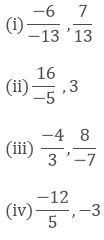Solution:Question: 4

Fill in the blanks by the correct symbol out of >, =, or < :Solution:

(i) Because every positive number is greater than a negative number, (- 6)/7 < 7/13.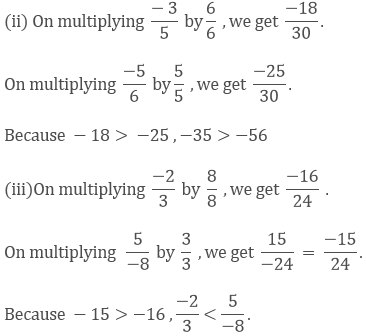(iv) Because every positive number is greater than a negative number, 0 > (-2)/5.

Question: 5

Arrange the following rational numbers in ascending order:Solution:

(i) Ascending order:

Since, LCM of 5, -30, -15, 10 is 30.

Multiplying the numerators and denominators to get the denominator equal to the LCM 3/5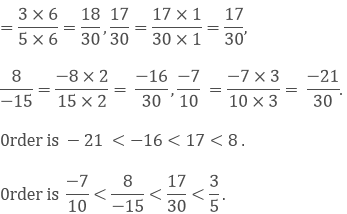(ii) Since, LCM of 9, -12, -18, 3 is 36.

Multiplying the numerators and denominators to get the denominator to get the denominator equal to the LCM ,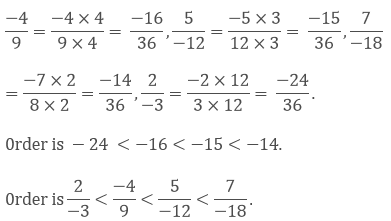Question: 6

Arrange the following rational numbers in descending order:Solution:

We have to arrange them in descending order.

(i) Since, LCM of 8, 16, -12, -4, 28 is 336.

Multiplying the numerators and denominators, to get the denominator equal to the LCM, 7/8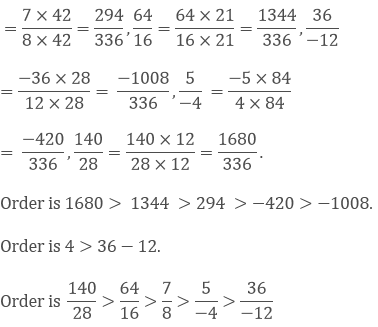(ii) Since, LCM of 10, -30, -15, 20 is 60.

Multiplying the numerators and denominators, to get the denominator equal to LCM,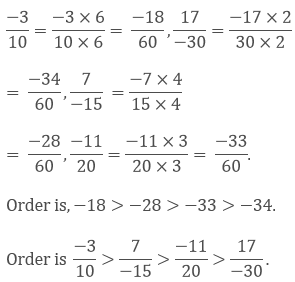Question: 7

Which of the following statements are true:

(i) The rational number 29/23 lies to the left of zero on the number line.

(ii) The rational number-12/-17 lies to the left of zero on the number line.

(iii) The rational number 3/4 lies to the right of zero on the number line.

(iv) The rational number (-12)/(-5) and (- 7)/(-17) are on the opposite side of zero on the number line.

(v) The rational number -2/15 and 7/(-31)  are on the opposite side of zero on the number line .

(vi) The rational number (- 3)/(-5)  is on the right of (- 4)/7 on the number line.

Solution:

(i) False; it lies to the right of zero because it is a positive number.

(ii) False; it lies to the right of zero because it is a positive number.

(iii) True

(iv) True; they are of opposite signs.

(v) False; they both are of same signs.

(vi) True; they both are of opposite signs and positive number is greater than the negative number.

Thus, it is on the right of the negative number.
```### Course Features

• 728 Video Lectures
• Revision Notes
• Previous Year Papers
• Mind Map
• Study Planner
• NCERT Solutions
• Discussion Forum
• Test paper with Video Solution